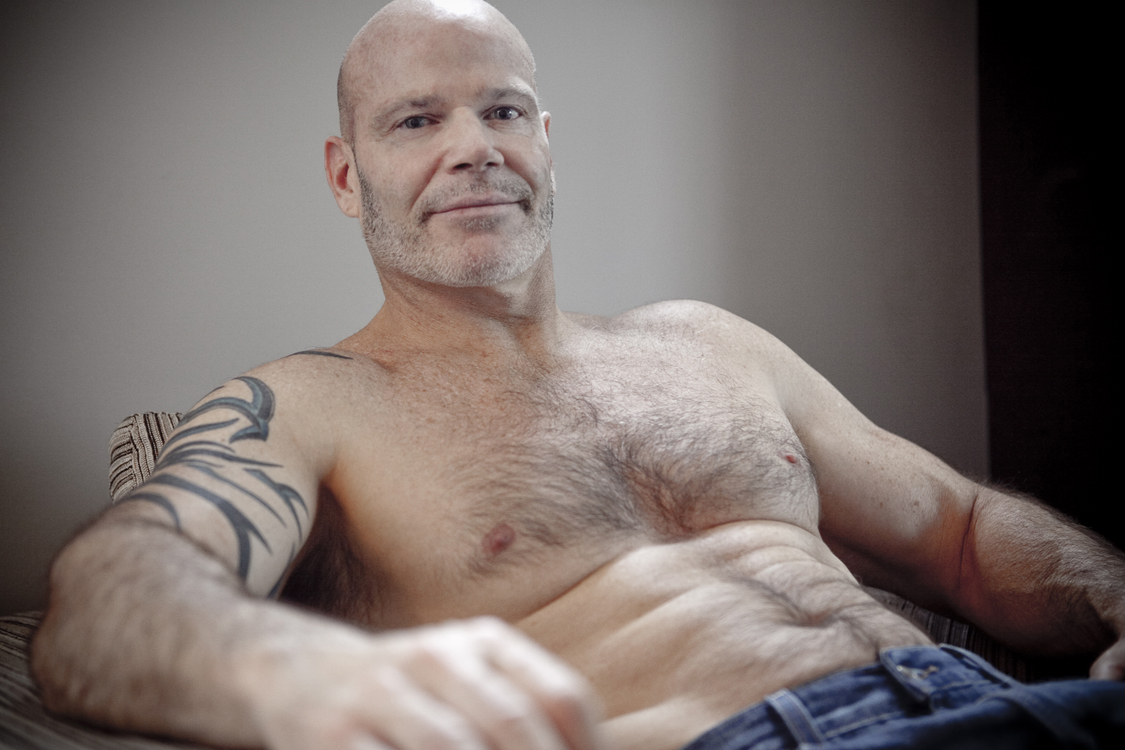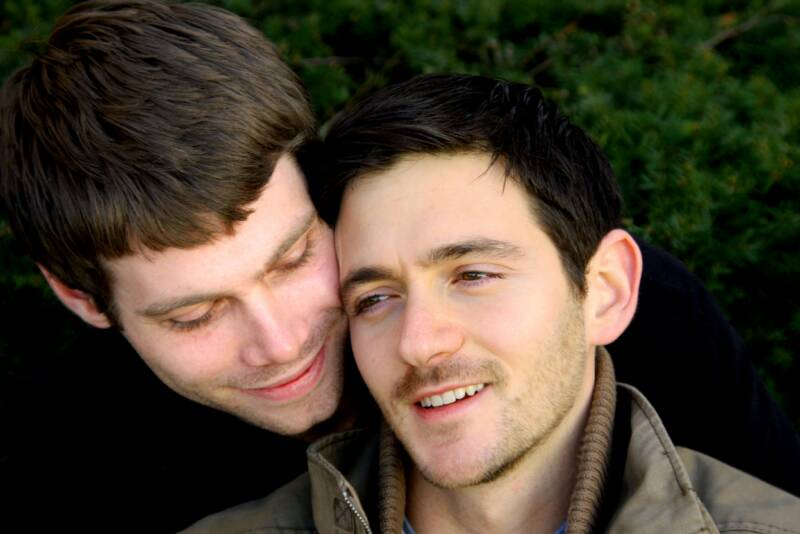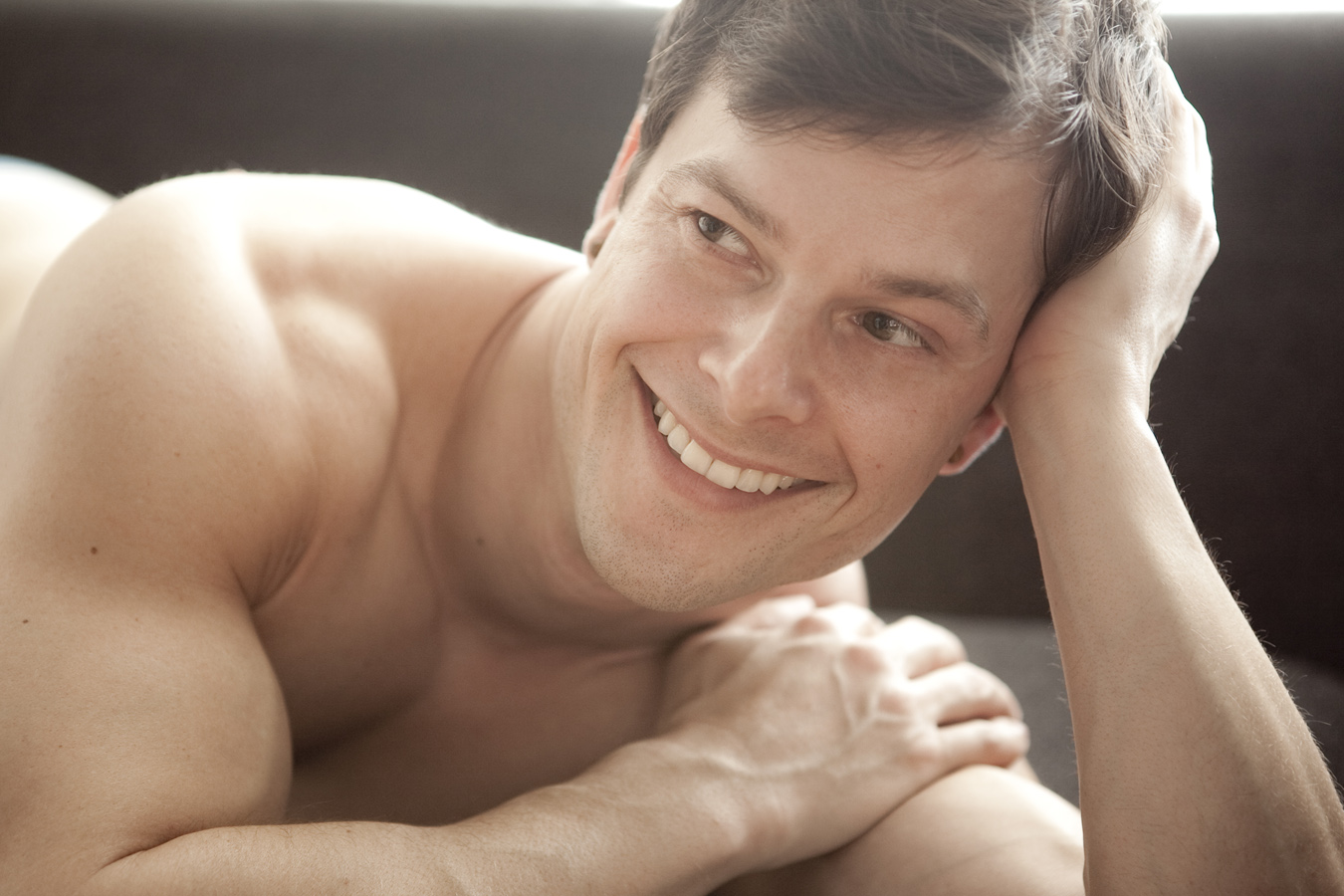A u s t i n
C a r l o s
J o e
H a o
M a r t a
H u n t e r
C h e n
J a k e
J e f  f
J o h n  V.
I v a n
K e v i n
R a f a e l e
R o c k d o g s
M e l i s s a
R i c h
C h r i s t o p h e r
R i c h  M.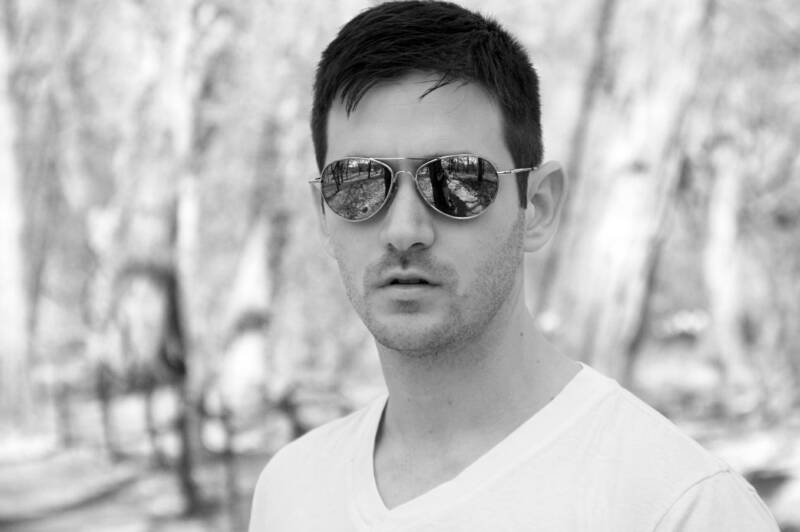All models were 18 years of age or older during the time of photography. All content and images are in full compliance with the requirements of 18 U.S.C. 2257 and associated regulations.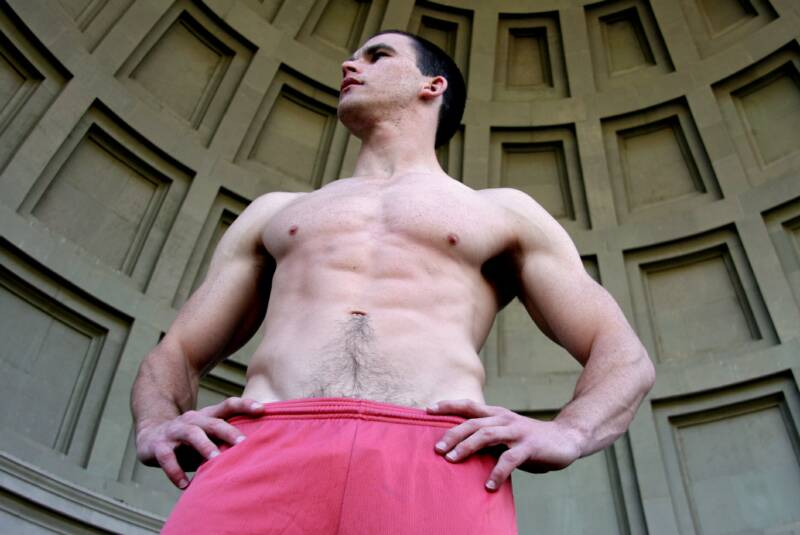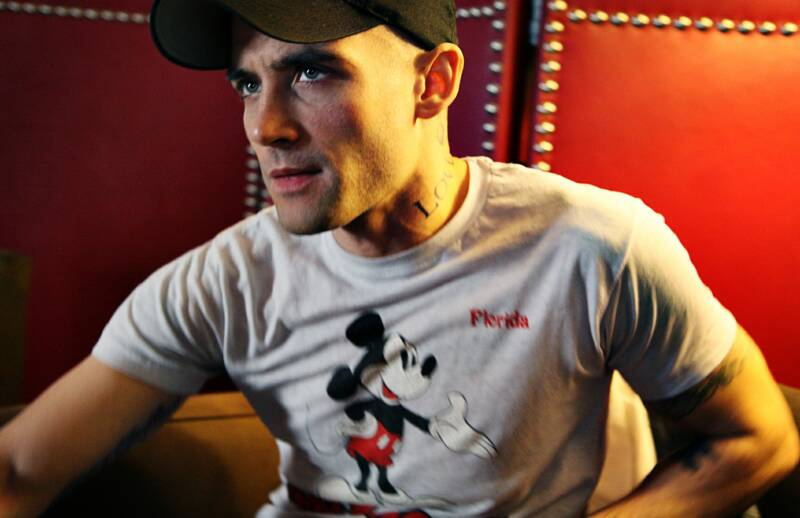N i c o l a s   S m i t h   P h o t o g r a p h y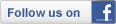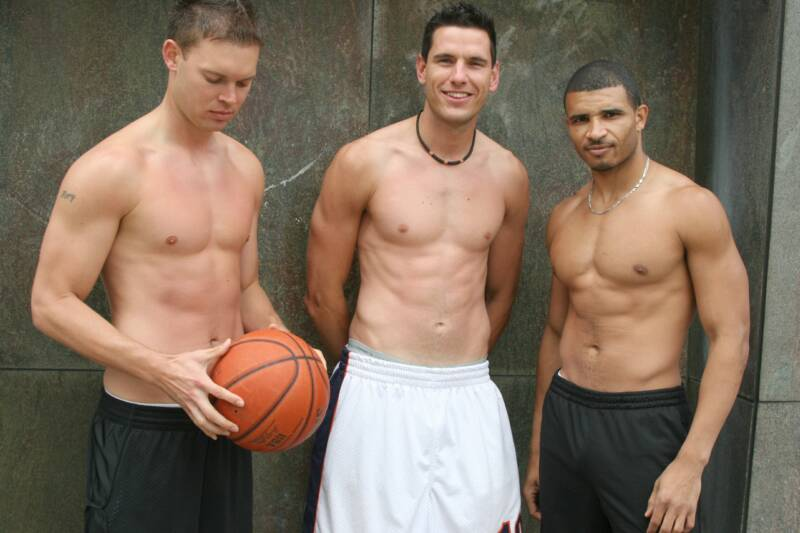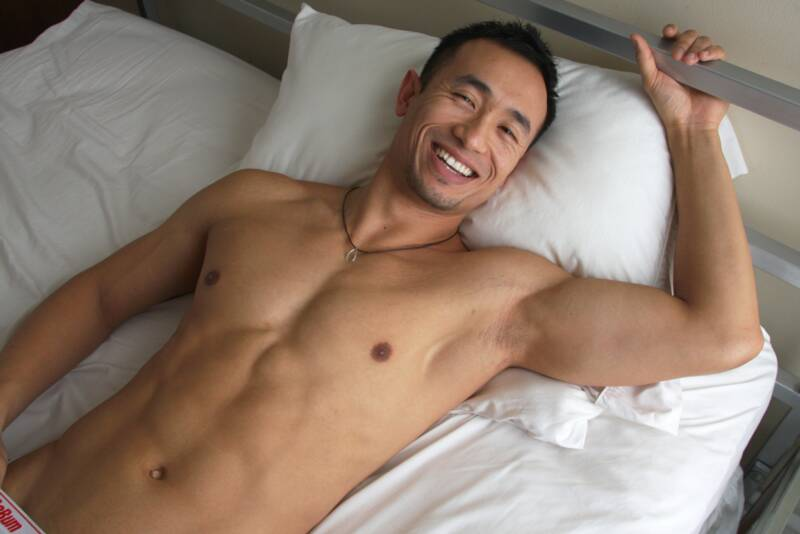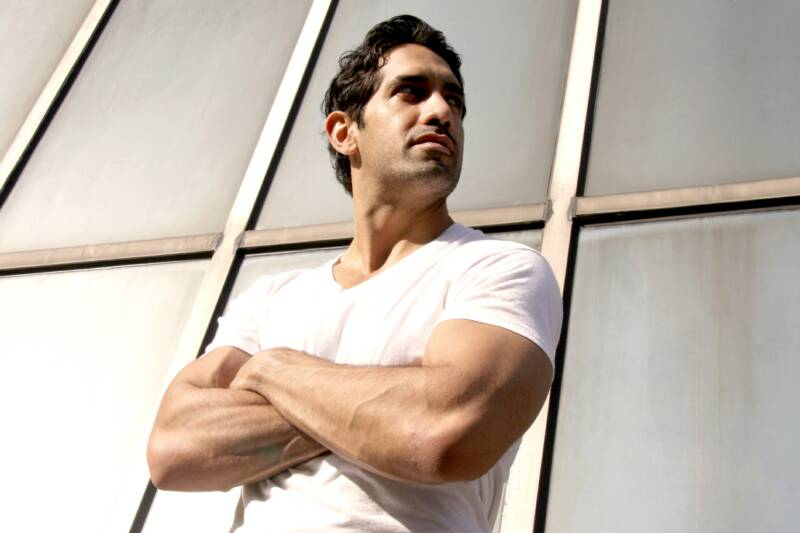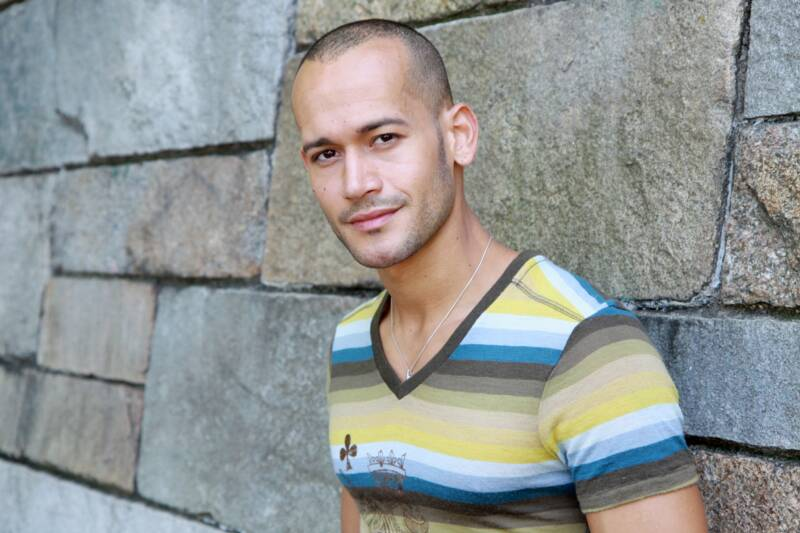Select a model to view their photos.  To book a photo shoot, click here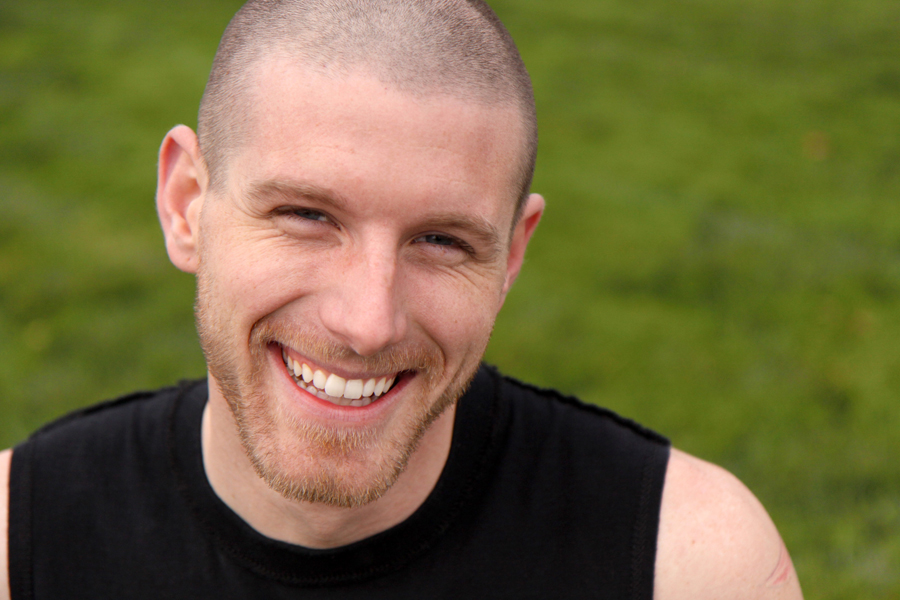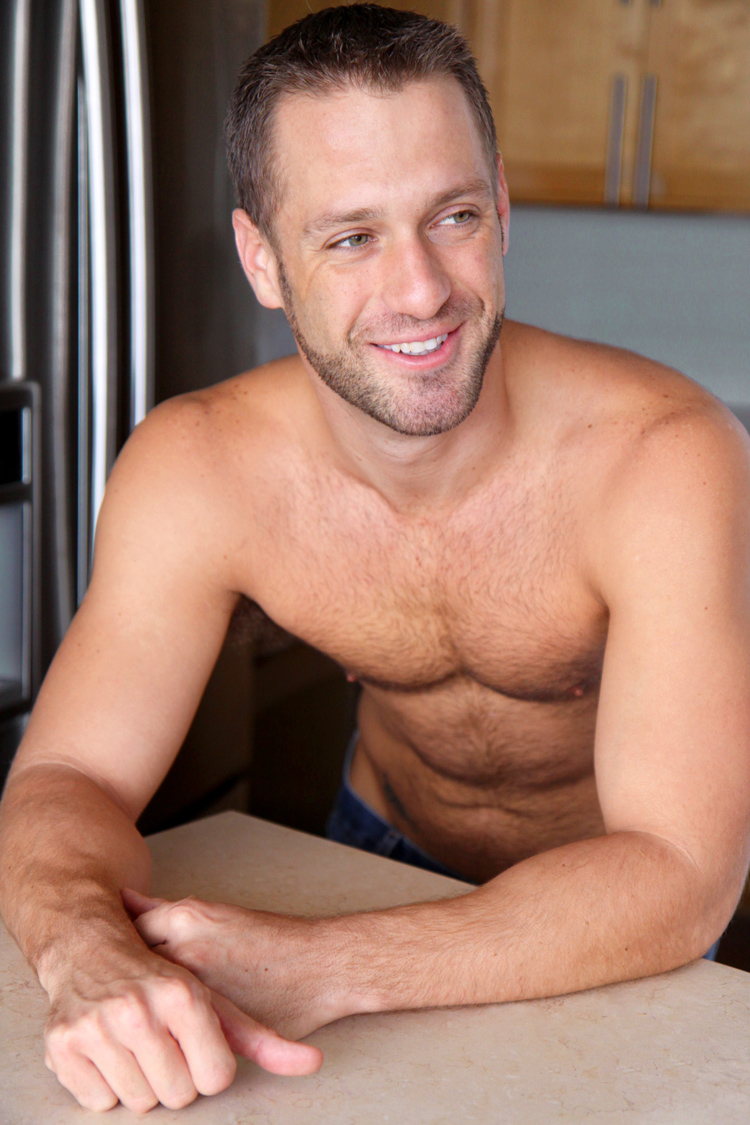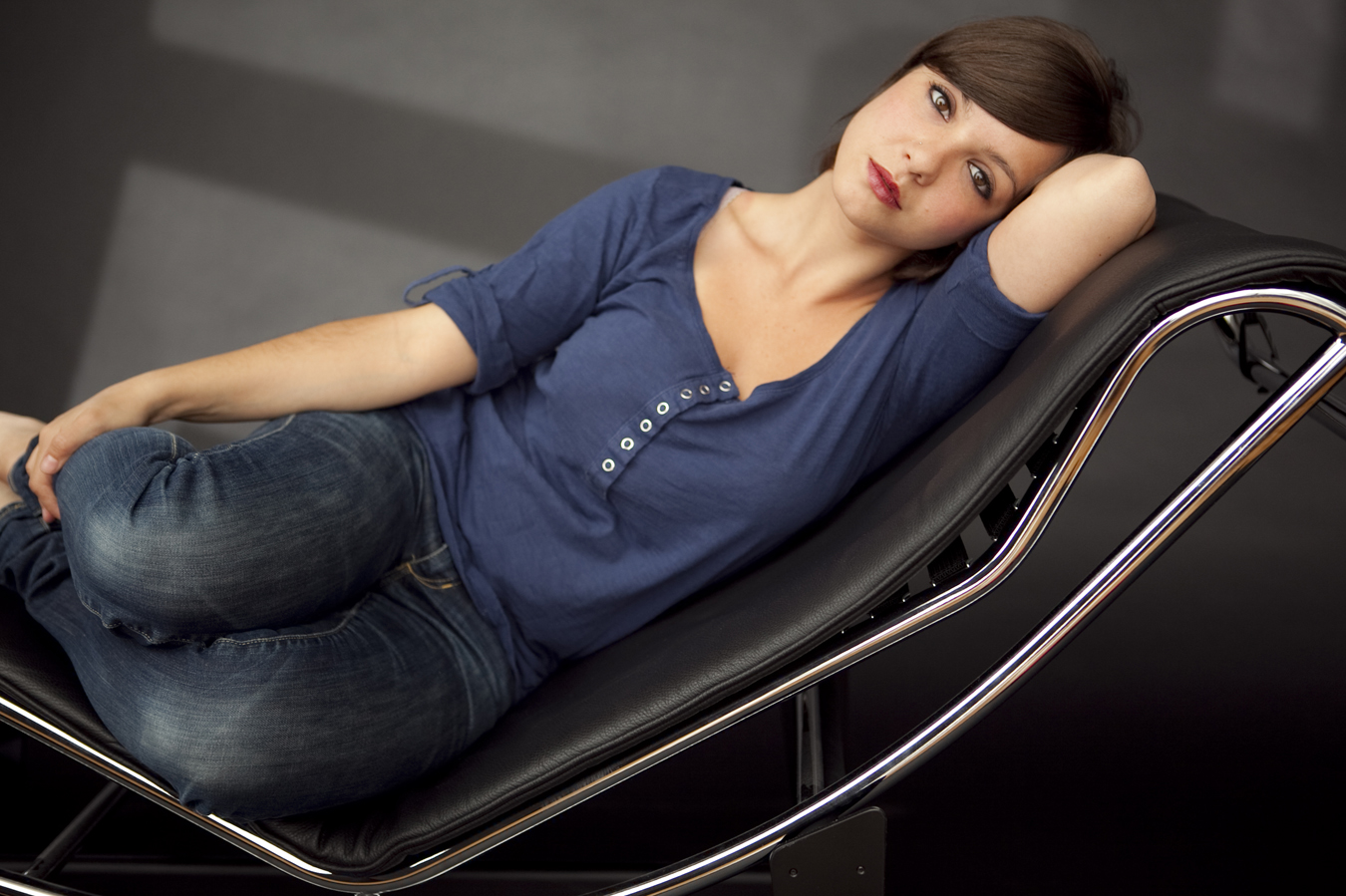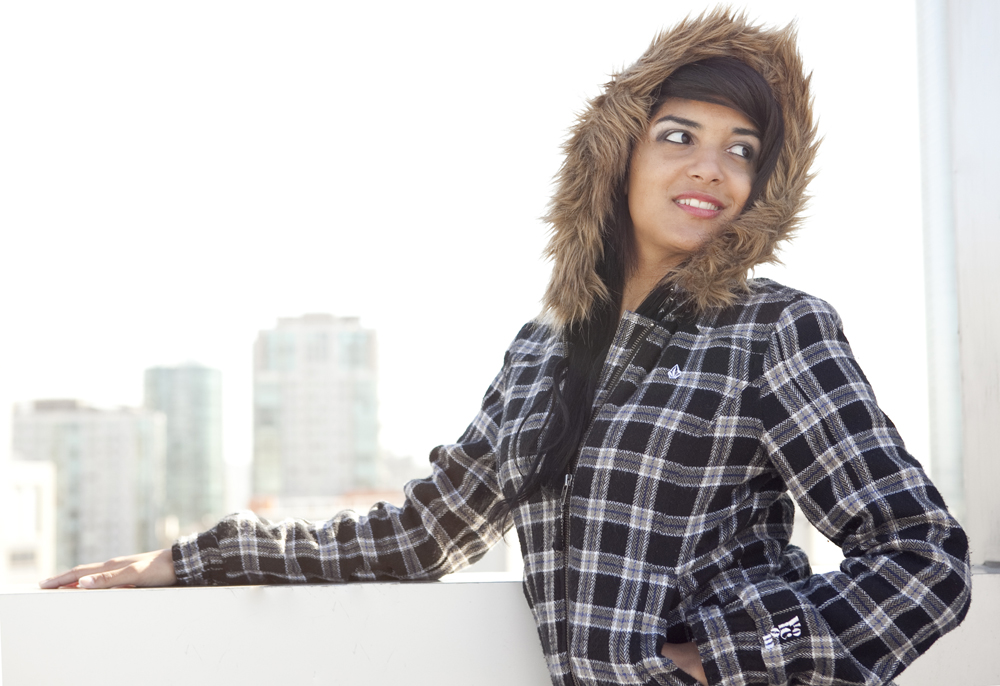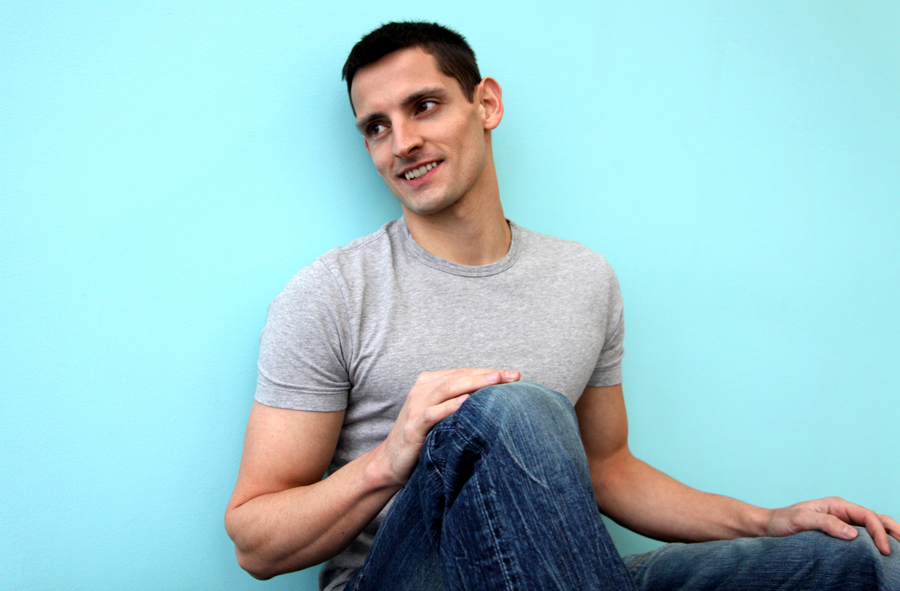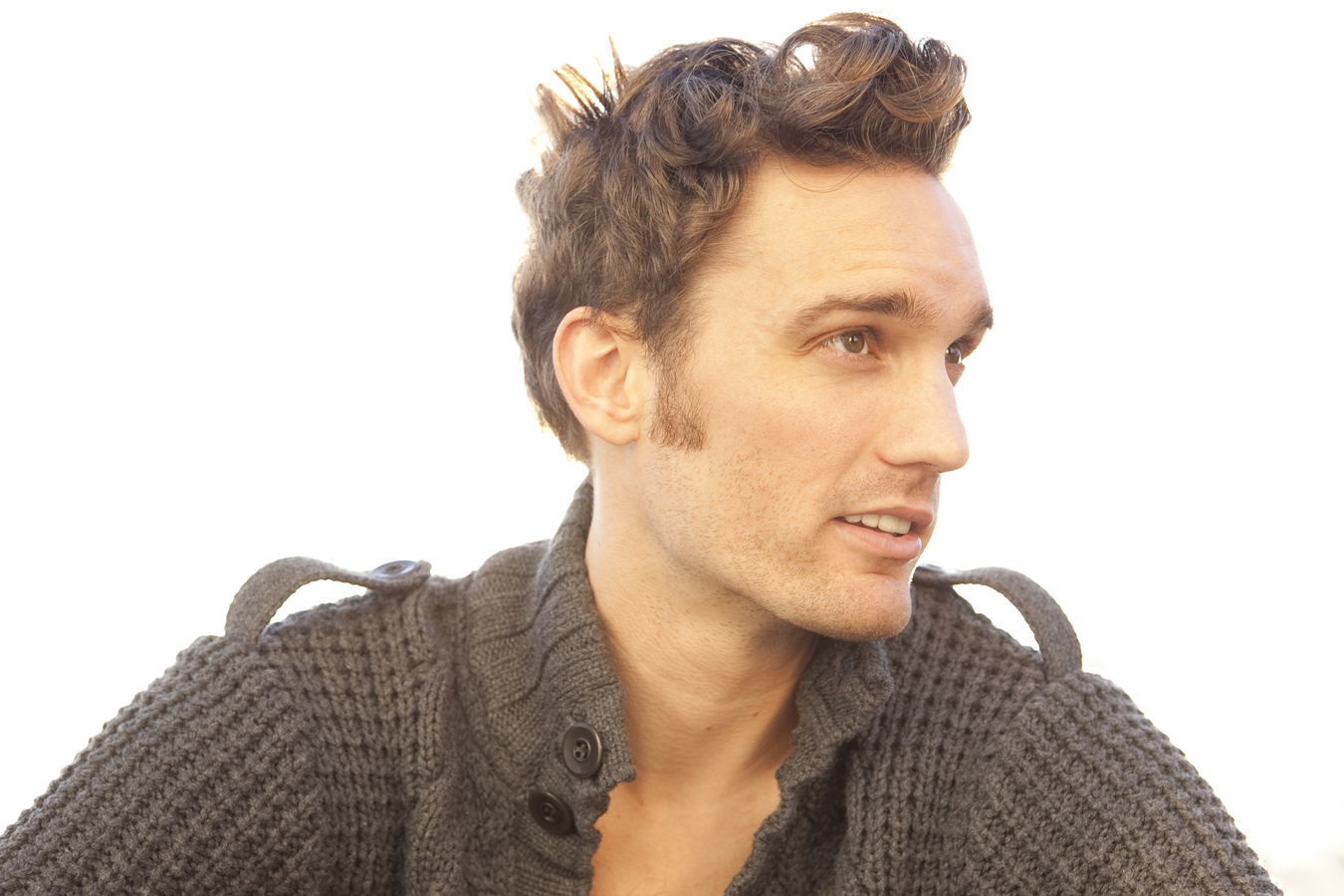S h a r o n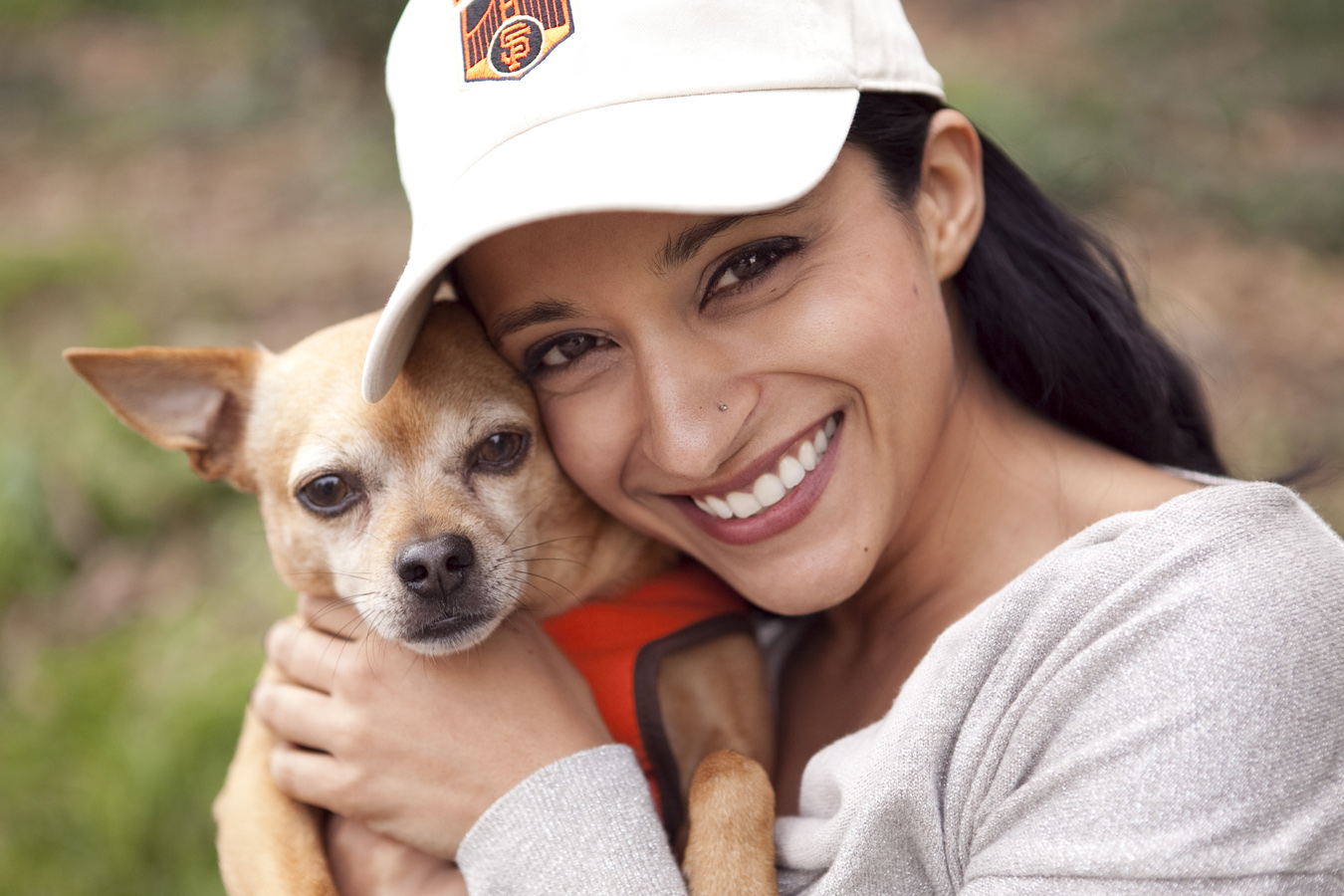R a n c e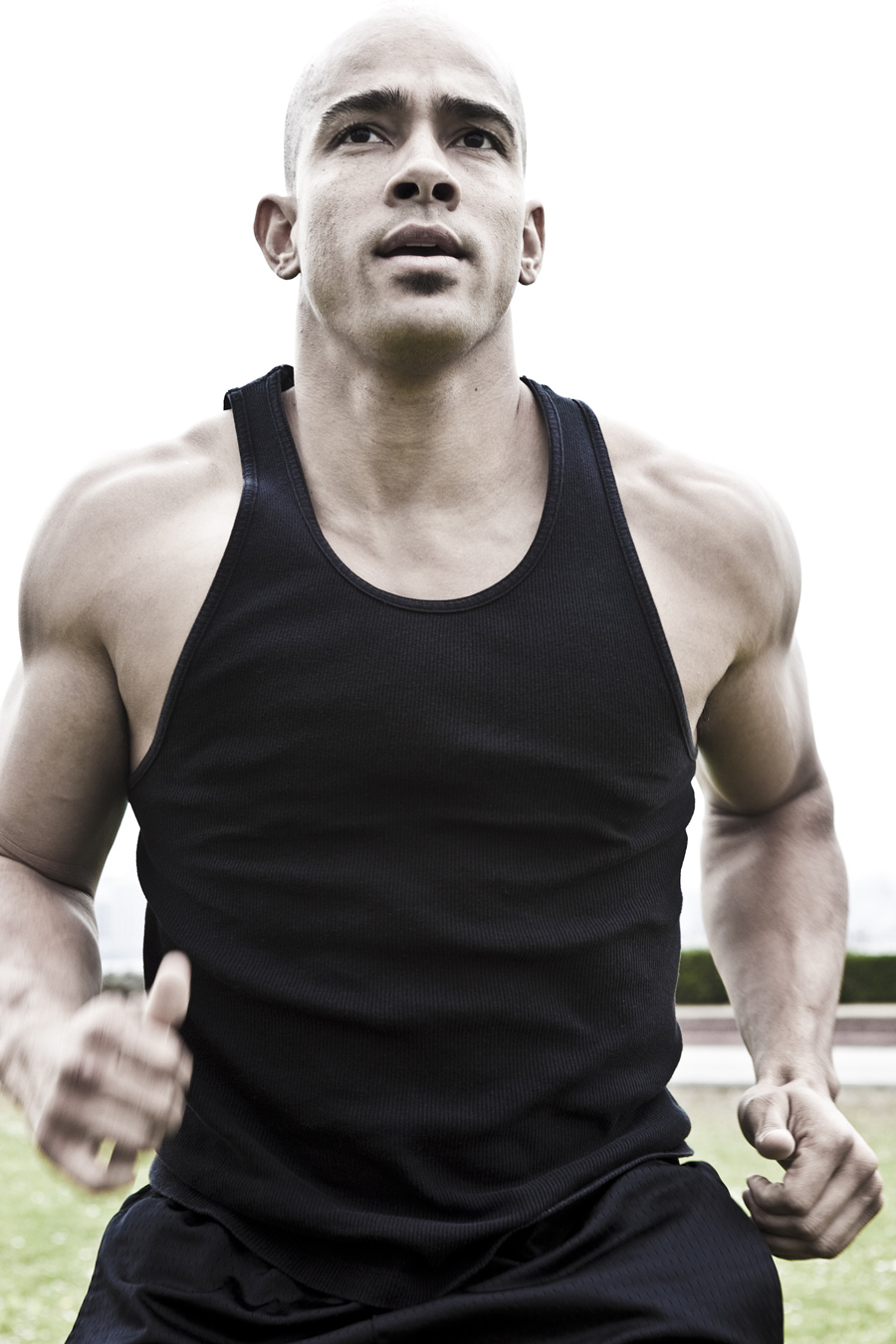Brett and Chen
A l e j a n d r o